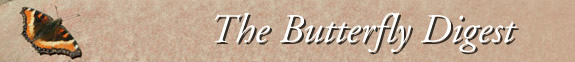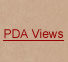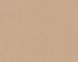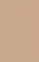Jump to : Today July 20, 2019 July 19, 2019 July 18, 2019 July 17, 2019 July 16, 2019 July 15, 2019 July 14, 2019 July 13, 2019 July 12, 2019 July 11, 2019 July 10, 2019 July 9, 2019 July 8, 2019 July 7, 2019 July 6, 2019 July 5, 2019 July 4, 2019 July 3, 2019 July 2, 2019 July 1, 2019 June 30, 2019 June 29, 2019 June 28, 2019 June 27, 2019 June 26, 2019 June 25, 2019 June 24, 2019 June 23, 2019 June 22, 2019 June 21, 2019     |    View All Lists    |    FAQ
Don,

My guess is a slightly =worn female Zabulon Skipper.

--Michael
=
On Fri=, Jun 21, 2019 at 3:04 PM donald adams <> wrote:

=E2=80=A6 at =E2=80=98Adams Farm=E2=80=99, West =Bridgewater =E2=80=A6

=C2=A0=

No id Skipper=C2=A0=C2=A0=C2=A0=C2=A0=C2==A0=C2=A0=C2=A0=C2=A0=C2=A0=C2=A0=C2=A0=C2=A0=C2=A0=C2=A0=C2=A0=C2=A0=C2=A0==C2=A0=C2=A0=C2=A0=C2=A0=C2=A0=C2=A0=C2=A0=C2=A0=C2=A0=C2=A0=C2=A0=C2=A0=C2==A0=C2=A0=C2=A0=C2=A0=C2=A0=C2=A0 1 (See Pic; HELP on id needed)<=/u>

Baltimore Checkersp=ot=C2=A0=C2=A0=C2=A0=C2=A0=C2=A0=C2=A0=C2=A0=C2=A0=C2=A0=C2=A0=C2=A0=C2=A0==C2=A0=C2=A0=C2=A0=C2=A0=C2=A0 1 (SOY; =E2=80=9CS=E2=80=9D for second out t=oday, Pic). First eclosure was on Wed 19 June, but from a chrysalis I had m=arked with a stake and watching for the past two weeks every day. So I actu=ally missed the butterfly and can only conclude from the remnant chorion it= eclosed a butterfly not a wasp.)

=C2=A0

Cool, rainy weather this week is causing =E2=80=9Cpe=nt-up eclosures=E2=80=99 so some butterflies popping out even on no-so-good= days, then hunkering down as best they can. This

weekend =C2=A0could be really gre=at for fresh sightings.

=C2=A0

--
You received this message because you are subscribed to the Google Groups &=quot;MassLep" group.
To unsubscribe from this group and stop receiving emails from it, send an e=mail to " target=3D"_=blank">masslep+.
To post to this group, send email to .